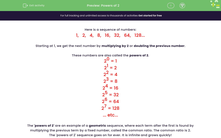# Understand the Powers of 2 Sequence

In this worksheet, students will work with the powers of 2 as an example of a geometric sequence.Key stage:  KS 3

Curriculum topic:   Algebra

Curriculum subtopic:   Use Geometric Sequences

Popular topics:   Algebra worksheets, Year 7 Algebra worksheets

Difficulty level:#### Worksheet Overview

Here is a sequence of numbers:

1,   2,   4,   8,   16,   32,   64,   128...

Starting at 1, we get the next number by multiplying by 2 or doubling the previous number.

These numbers are also called the powers of 2.

20 = 1

21 = 2

22 = 4

23 = 8

24 = 16

25 = 32

26 = 64

27 = 128

... etc...

The 'powers of 2' are an example of a geometric sequence, where each term after the first is found by multiplying the previous term by a fixed number, called the common ratio. The common ratio is 2.

The 'powers of 2' sequence goes on for ever. It is infinite and grows quickly!Let's have a go at some questions now,

### What is EdPlace?

We're your National Curriculum aligned online education content provider helping each child succeed in English, maths and science from year 1 to GCSE. With an EdPlace account you’ll be able to track and measure progress, helping each child achieve their best. We build confidence and attainment by personalising each child’s learning at a level that suits them.

Get started